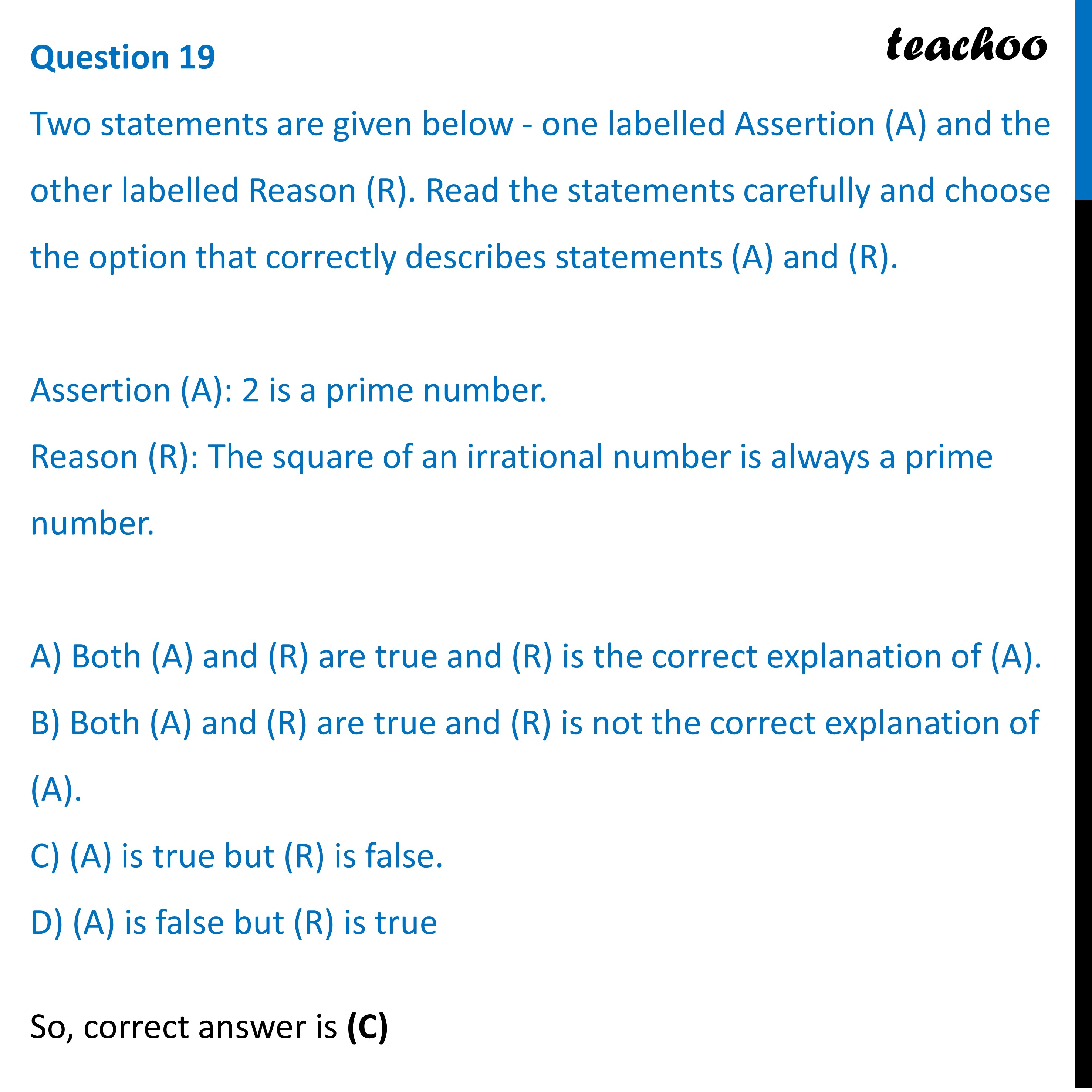Practice Questions CBSE - Maths Class 10 (2023 Boards)

Class 10
Solutions of Sample Papers for Class 10 Boards

## D) (A) is false but (R) is trueLearn in your speed, with individual attention - Teachoo Maths 1-on-1 Class

### Transcript

Two statements are given below - one labelled Assertion (A) and the other labelled Reason (R). Read the statements carefully and choose the option that correctly describes statements (A) and (R). Assertion (A): 2 is a prime number. Reason (R): The square of an irrational number is always a prime number. A) Both (A) and (R) are true and (R) is the correct explanation of (A). B) Both (A) and (R) are true and (R) is not the correct explanation of (A). C) (A) is true but (R) is false. D) (A) is false but (R) is true